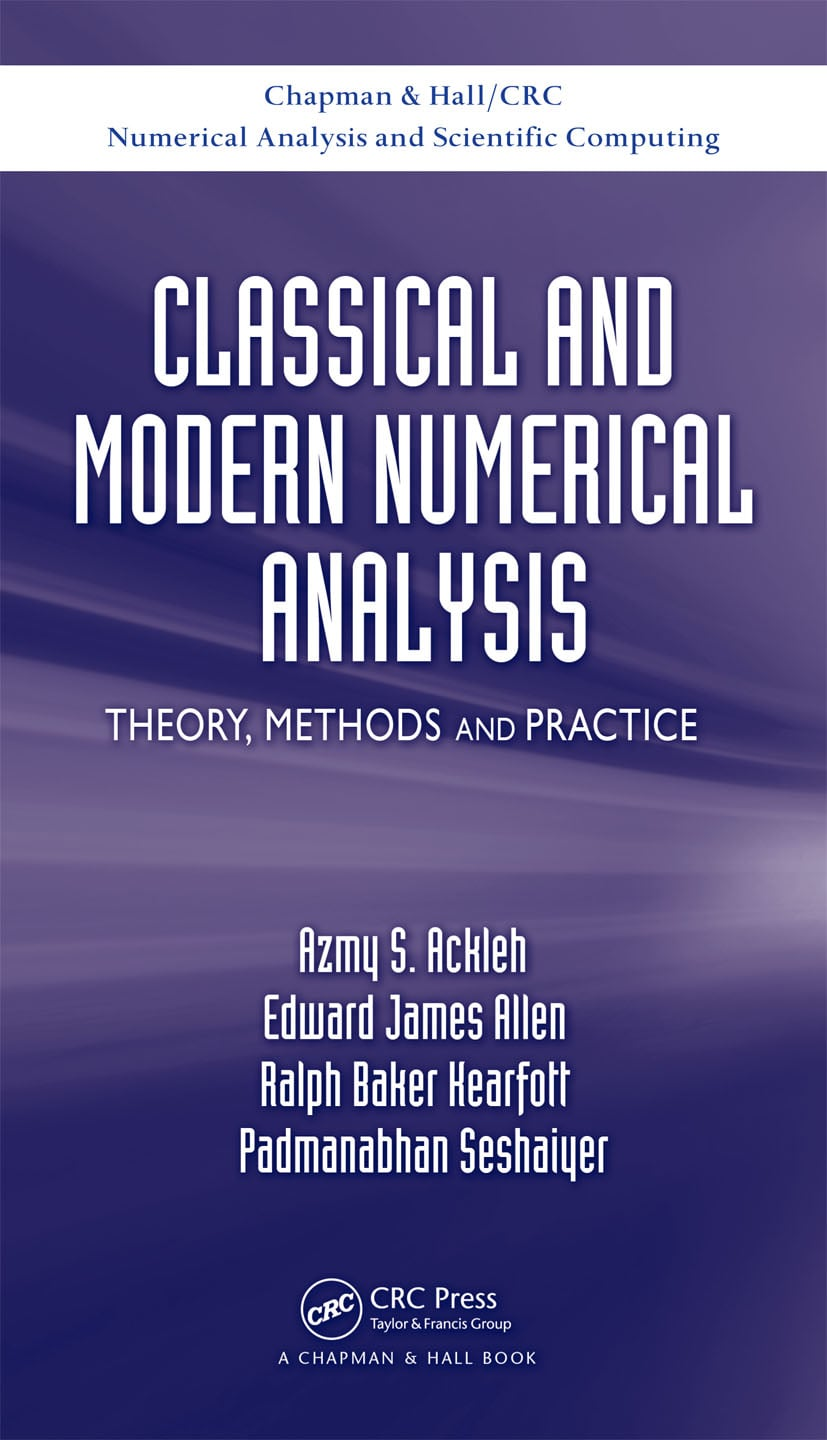Classical and Modern Numerical Analysis

Theory, Methods and Practice, 1st Edition

Chapman and Hall/CRC

628 pages | 40 B/W Illus.

Purchasing Options:\$ = USD
Hardback: 9781420091571
pub: 2009-07-20
SAVE ~\$28.00
\$140.00
\$112.00
x
eBook (VitalSource) : 9780429142062
pub: 2009-07-20
from \$70.00

FREE Standard Shipping!

Description

Classical and Modern Numerical Analysis: Theory, Methods and Practice provides a sound foundation in numerical analysis for more specialized topics, such as finite element theory, advanced numerical linear algebra, and optimization. It prepares graduate students for taking doctoral examinations in numerical analysis.

The text covers the main areas of introductory numerical analysis, including the solution of nonlinear equations, numerical linear algebra, ordinary differential equations, approximation theory, numerical integration, and boundary value problems. Focusing on interval computing in numerical analysis, it explains interval arithmetic, interval computation, and interval algorithms. The authors illustrate the concepts with many examples as well as analytical and computational exercises at the end of each chapter.

This advanced, graduate-level introduction to the theory and methods of numerical analysis supplies the necessary background in numerical methods so that students can apply the techniques and understand the mathematical literature in this area. Although the book is independent of a specific computer program, MATLAB® code is available on the authors' website to illustrate various concepts.

Reviews

…this book provides useful background knowledge for graduate study in any area of applied mathematics … this is a thorough, well-written treatment of an important subject.

Computing Reviews, May 2010

Mathematical Review and Computer Arithmetic

Mathematical Review

Computer Arithmetic

Interval Computations

Numerical Solution of Nonlinear Equations of One Variable

Introduction

Bisection Method

The Fixed Point Method

Newton’s Method (Newton–Raphson Method)

The Univariate Interval Newton Method

Secant Method and Müller’s Method

Aitken Acceleration and Steffensen’s Method

Roots of Polynomials

Additional Notes and Summary

Numerical Linear Algebra

Basic Results from Linear Algebra

Normed Linear Spaces

Direct Methods for Solving Linear Systems

Iterative Methods for Solving Linear Systems

The Singular Value Decomposition

Approximation Theory

Introduction

Norms, Projections, Inner Product Spaces, and Orthogonalization in Function Spaces

Polynomial Approximation

Piecewise Polynomial Approximation

Trigonometric Approximation

Rational Approximation

Wavelet Bases

Least Squares Approximation on a Finite Point Set

Eigenvalue-Eigenvector Computation

Basic Results from Linear Algebra

The Power Method

The Inverse Power Method

Deflation

The QR Method

Jacobi Diagonalization (Jacobi Method)

Simultaneous Iteration (Subspace Iteration)

Numerical Differentiation and Integration

Numerical Differentiation

Automatic (Computational) Differentiation

Numerical Integration

Initial Value Problems for Ordinary Differential Equations

Introduction

Euler’s Method

Single-Step Methods: Taylor Series and Runge–Kutta

Error Control and the Runge–Kutta–Fehlberg Method

Multistep Methods

Predictor-Corrector Methods

Stiff Systems

Extrapolation Methods

Application to Parameter Estimation in Differential Equations

Numerical Solution of Systems of Nonlinear Equations

Introduction and Fréchet Derivatives

Successive Approximation (Fixed Point Iteration) and the Contraction Mapping Theorem

Newton’s Method and Variations

Multivariate Interval Newton Methods

Quasi-Newton Methods (Broyden’s Method)

Methods for Finding All Solutions

Optimization

Local Optimization

Constrained Local Optimization

Constrained Optimization and Nonlinear Systems

Linear Programming

Dynamic Programming

Global (Nonconvex) Optimization

Boundary-Value Problems and Integral Equations

Boundary-Value Problems

Approximation of Integral Equations

Appendix: Solutions to Selected Exercises

References

Index

Exercises appear at the end of each chapter.

Azmy S. Ackleh is Dr. Ray P. Authement/BORSF Eminent Scholar Endowed Chair in Computational Mathematics at the University of Louisiana. Dr. Ackleh has more than fifteen years experience in mathematical biology with an emphasis on the long-time behavior of discrete and continuous population models and numerical methods for structured-population models.

Edward James Allen is a professor of mathematics at Texas Tech University. Dr. Allen works primarily on the derivation and computation of stochastic differential equation models in biology and physics and on the development and analysis of numerical methods for problems in neutron transport.

R. Baker Kearfott is a professor of mathematics at the University of Louisiana, with over thirty years experience teaching numerical analysis. Dr. Kearfott’s research focuses on nonlinear equations, nonlinear optimization, and mathematically rigorous numerical analysis.

Padmanabhan Seshaiyer is an associate professor of mathematical sciences at George Mason University. Dr. Seshaiyer has done extensive work on the theoretical and computational aspects of finite element methods and applications of numerical methods to biological and bio-inspired problems.